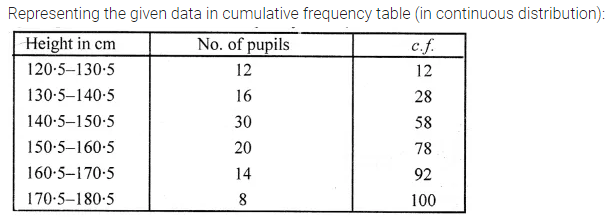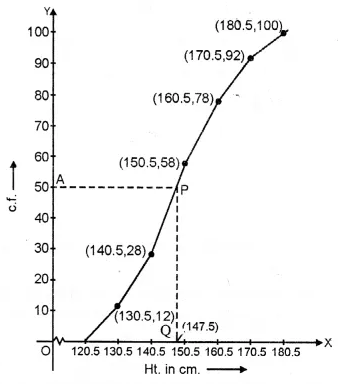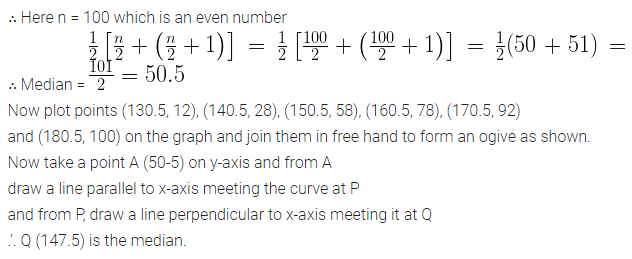# ML Aggarwal Class 10 Solutions for ICSE Maths Chapter 21 Measures of Central Tendency Ex 21.6

## ML Aggarwal Class 10 Solutions for ICSE Maths Chapter 21 Measures of Central Tendency Ex 21.6

ML Aggarwal Class 10 Solutions for ICSE Maths Chapter 21 Measures of Central Tendency Ex 21.6

Question 1.
The following table shows the distribution of the heights of a group of factory workers.(i) Determine the cumulative frequencies.
(ii) Draw the cumulative frequency curve on a graph paper.
Use 2 cm = 5 cm height on one axis and 2 cm = 10 workers on the other.
(iii) From your graph, write down the median height in cm.
Solution: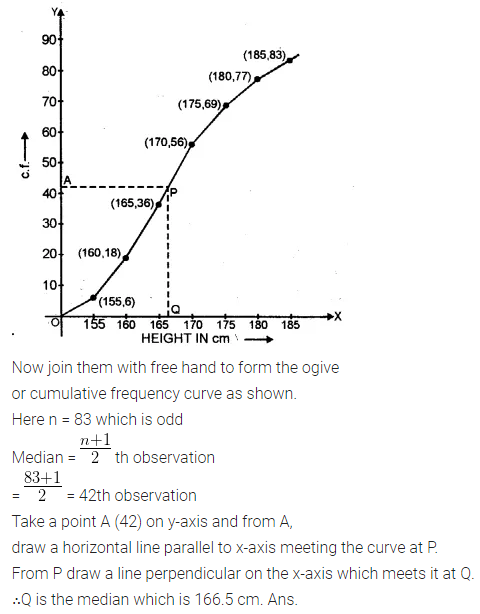Question 2.
Using the data given below construct the cumulative frequency table and draw the-Ogive. From the ogive determine the median.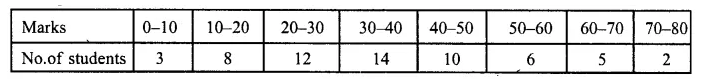Solution:Question 3.
Use graph paper for this question.
The following table shows the weights in gm of a sample of 100 potatoes taken from a large consignment:(i) Calculate the cumulative frequencies.
(ii) Draw the cumulative frequency curve and from it determine the median weight of the potatoes. (1996)
Solution:Question 4.
Attempt this question on graph paper.(i) Construct the ‘less than’ cumulative frequency curve for the above data, using 2 cm = 10 years, on one axis and 2 cm = 10 casualties on the other.
(ii) From your graph determine (1) the median and (2) the upper quartile
Solution: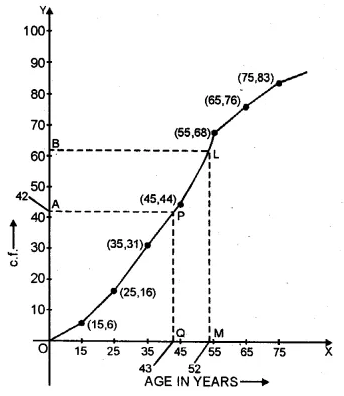Question 5.
The weight of 50 workers is given below: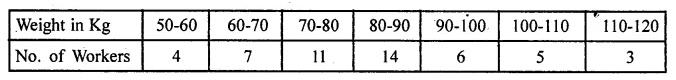Draw an ogive of the given distribution using a graph sheet. Take 2 cm = 10 kg on one axis, and 2 cm = 5 workers along the other axis. Use a graph to estimate the following:
(i) the upper and lower quartiles.
(ii) if weighing 95 kg and above is considered overweight find the number of workers who are overweight. (2015)
Solution: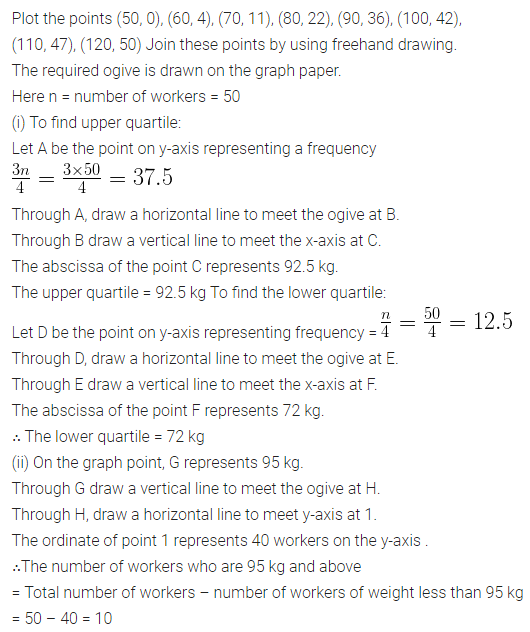Question 6.
The table shows the distribution of scores obtained by 160 shooters in a shooting competition. Use a graph sheet and draw an ogive for the distribution.
(Take 2 cm = 10 scores on the x-axis and 2 cm = 20 shooters on the y-axis)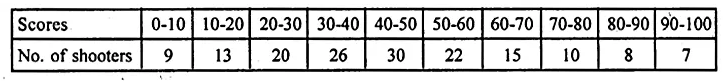Use your graph to estimate the following:
(i) The median.
(ii) The interquartile range.
(iii) The number of shooters who obtained a score of more than 85%.
Solution: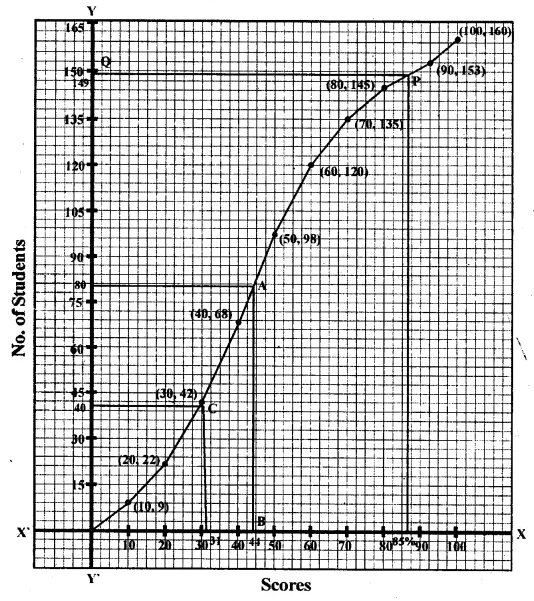Question 7.
The daily wages of 80 workers in a project are given belowUse a graph paper to draw an ogive for the above distribution.
(Use a scale of 2 cm = Rs 50 on x-axis and 2 cm = 10 workers on y-axis).
(i) the median wage of the workers.
(ii) the lower quartile wage of the workers.
(iii) the number of workers who earn more than Rs 625 daily. (2017)
Solution: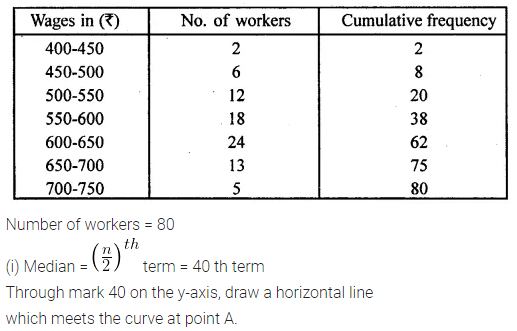Question 8.
Marks obtained by 200 students in an examination are given below :Draw an ogive for the given distribution taking 2 cm = 10 marks on one axis and 2 cm = 20 students on the other axis. Using the graph, determine
(i) The median marks.
(ii) The number of students who failed if minimum marks required to pass is 40.
(iii) If scoring 85 and more marks are considered as grade one, find the number of students who secured grade one in the examination.
Solution:Question 9.
The monthly income of a group of 320 employees in a company is given below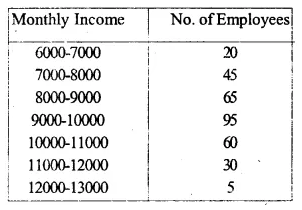Draw an ogive of the given distribution on a graph sheet taking 2 cm = Rs. 1000 on one axis and 2 cm = 50 employees on the other axis. From the graph determine
(i) the median wage.
(ii) the number of employees whose income is below Rs. 8500.
(iii) If the salary of a senior employee is above Rs. 11500, find the number of senior employees in the company.
(iv) the upper quartile.
Solution: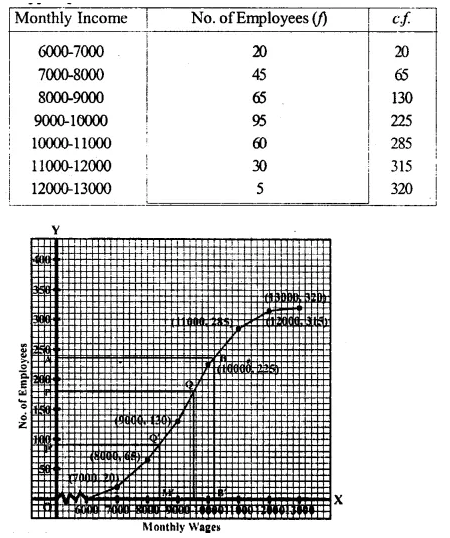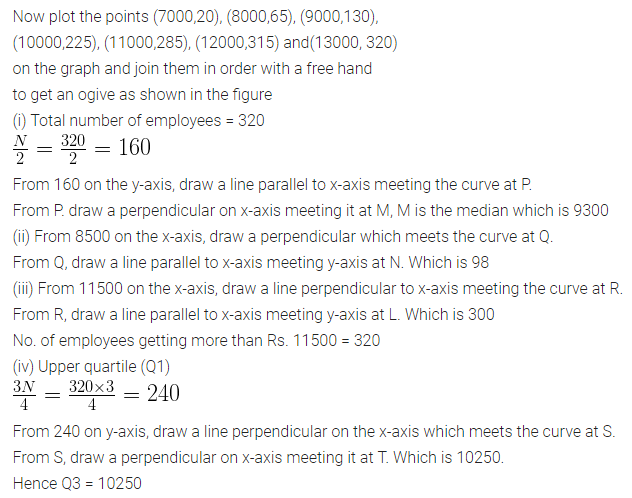Question 10.
Using a graph paper, draw an ogive for the following distribution which shows a record of the weight in kilograms of 200 studentsUse your ogive to estimate the following:
(i) The percentage of students weighing 55 kg or more.
(ii) The weight above which the heaviest 30% of the students fall,
(iii) The number of students who are :
1. under-weight and
2. over-weight, if 55.70 kg is considered as standard weight.
Solution: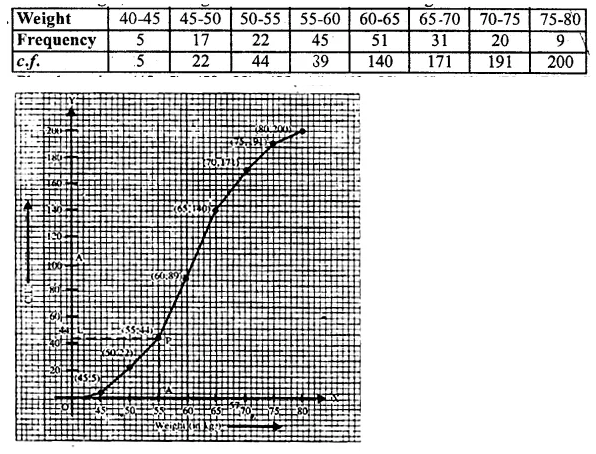Question 11.
The marks obtained by 100 students in a Mathematics test are given below :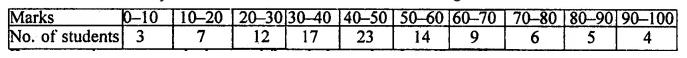Draw an ogive on a graph sheet and from it determine the :
(i) median
(ii) lower quartile
(iii) number of students who obtained more than 85% marks in the test.
(iv) number of students who did not pass in the test if the pass percentage was 35. We represent the given data in cumulative frequency table as given below :
Solution: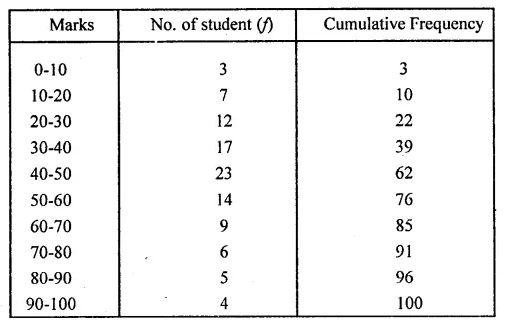Question 12.
The marks obtained by 120 students in a Mathematics test are given belowDraw an ogive for the given distribution on a graph sheet. Use a suitable scale for ogive to estimate the following:
(i) the median
(ii) the lower quartile
{iii) the number of students who obtained more than 75% marks in the test.
(iv) the number of students who did not pass in the test if the pass percentage was 40. (2002)
Solution: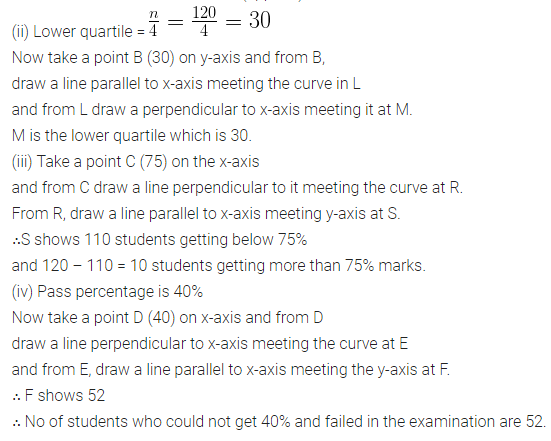Question 13.
The following distribution represents the height of 160 students of a school.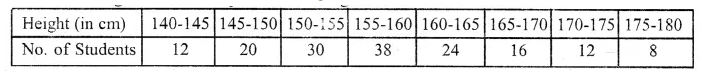Draw an ogive for the given distribution taking 2 cm = 5 cm of height on one axis and 2 cm = 20 students on the other axis. Using the graph, determine :
(i)The median height.
(ii)The interquartile range.
(iii) The number of students whose height is above 172 cm.
Solution: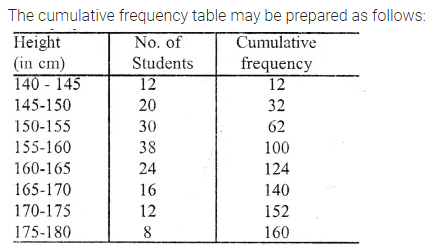Question 14.
100 pupils in a school have heights as tabulated below :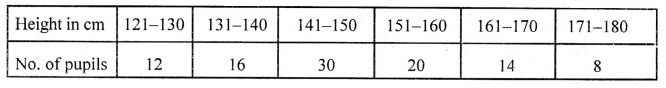Draw the ogive for the above data and from it determine the median (use graph paper).
Solution: